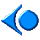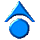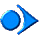# ThreadMentor: Managing a Shared Counter### Problem

Suppose we have two groups of threads and a shared counter. Threads in the first group increase the value of the shared counter by 1 repeatedly, while threads in the second group decreases the counter's value by 1. This can easily be done with a single Mutex lock. However, this requires every thread to follow the same lock-unlock protocol, and, if one of these thread does it wrong, mutual exclusion cannot be guaranteed. Let us do it using a monitor.

### Analysis

Since mutual exclusion of a monitor is established by methods MonitorBegin() and MonitorEnd(), we can move the shared counter into a monitor as a private data item, and design monitor procedures Increment() to increase the counter by 1 and Decrement() to decrease the counter value by 1.

### Program

Our monitor definition is shown below. The name of this monitor is CounterMonitor. It has a constructor, two public monitor procedures Increment() and Decrement(), and a private variable counter. Just like threads we have the monitor definition in a header .h file and its implementation in a .cpp file with the same name. Therefore, the monitor definition is in the file IncDec-mon.h.

 ```#include "ThreadClass.h" class CounterMonitor : public Monitor { public: CounterMonitor(char* Name); // constructor int Increment(); // increment the counter int Decrement(); // decrement the counter private: int counter; // internal counter }; ``` Click here to download this file (IncDec-mon.h)

Let use see how the monitor procedures are implemented. The constructor is simple. It sets the monitor name, makes the monitor of Hoare style (i.e., argument HOARE), and clears variable counter. Monitor procedure Increment() first locks the monitor by calling MonitorBegin(). Once the monitor is locked (i.e., the calling thread is in the monitor), the counter value can be safely increased. Note that the new value is saved in a local variable number so that it can be returned to the calling thread properly. After this and before leaving the monitor, method MonitorEnd() is called to unlock the monitor for other threads to get in. Finally, the value of the local variable number is returned. Note that this is the value after this thread's increment. The monitor procedure Decrement() is similar and will not be discussed. The two procedures and the constructor are saved in a file IncDec-mon.cpp as shown below. Now, our monitor implementation completes.

 ```#include #include "ThreadClass.h" #include "IncDec-mon.h" CounterMonitor::CounterMonitor(char* Name) : Monitor(Name, HOARE) { counter = 0; // clear the counter } int CounterMonitor::Increment() { int number; MonitorBegin(); // lock the monitor counter++; // increase the counter number = counter; // save its value MonitorEnd(); // release the monitor return number; // return the counter value } int CounterMonitor::Decrement() { int number; MonitorBegin(); // lock the monitor counter--; // decrease the counter number = counter; // save its value MonitorEnd(); // release the monitor return number; // return the counter value } ``` Click here to download this file (IncDec-mon.cpp)

We should see how threads can use these two monitor procedures. The thread class is simple. The char variable Id is used to distinguish if a thread will increase the counter. This status is assigned by the main program when threads are created.

 ```#include "ThreadClass.h" class IncDecThread: public Thread { public: IncDecThread(int No, char ID); // constructor private: void ThreadFunc(); int number; char Id; // Inc or Dec key }; ``` Click here to download this file (IncDec-Thrd.h)

The thread itself is also simple. It loops for NUM_OF_TIMES iterations. For each iteration, based on the assigned role, it either increases the counter value by calling method Counter.Increment() or decreases the counter value by calling method Counter.Decrement(). Then, the new value after an operation is performed on the counter is printed. It is clear from this example that, compared with the use of semaphores, the use of monitor makes our code more structured and centralized.

 ```#include #include "ThreadClass.h" #include "IncDec-Thrd.h" #include "IncDec-mon.h" #define NUM_OF_TIMES 10 // maximum number of iterations // static data variables static CounterMonitor Counter("CounterMonitor"); strstream *Filler(int n) { int i; strstream *Space; Space = new strstream; for (i = 0; i < n; i++) (*Space) << ' '; (*Space) << '\0'; return Space; } IncDecThread::IncDecThread(int No, char ID) : number(No), Id(ID) { ThreadName.seekp(0, ios::beg); if (ID == 'I') // an increment thread ThreadName << "IncThread" << No << '\0'; else ThreadName << "DecThread" << No << '\0'; } void IncDecThread::ThreadFunc() { Thread::ThreadFunc(); int localCounter; strstream *Space; Space = Filler(this->number); for (int i = 1; i <= NUM_OF_TIMES; i++) { Delay(); if (Id == 'I') { // increase the counter localCounter = Counter.Increment(); cout << Space->str() << ThreadName.str() << " increment the counter to " << localCounter << endl; } else { // decrease the counter localCounter = Counter.Decrement(); cout << Space->str() << ThreadName.str() << " decrement the counter to " << localCounter << endl; } } cout << Space->str() << ThreadName.str() << " Exit" << endl; Exit(); } ``` Click here to download this file (IncDec-Thrd.cpp)

The main program creates a number of threads. Before a thread is created, the main program uses a random number to determine if this thread is of an increment type or a decrement type. After a thread is created, it is run. After all threads are created, the main program waits for the completion of all threads.

 ```#include #include #include #include "ThreadClass.h" #include "IncDec-Thrd.h" #include "IncDec-mon.h" #define MAX_NUM 20 // maximum number of inc/dec threads int main(int argc, char *argv[]) { IncDecThread *thread[MAX_NUM]; int NumberOfIncThreads = 0, // number of increment threads NumberOfDecThreads = 0; // number of decrement threads int NumberOfThreads; // total number of threads int i; if (argc != 2) { // verify user input cout << "Usage " << argv << " #-of-Threads" << endl; exit(0); } else NumberOfThreads = abs(atoi(argv)); srand((unsigned int) time(NULL)); // initialize random seed // create the increment/decrement threads in a random way for (i = 0; i < NumberOfThreads; i++) { // create threads if (rand() % 10 <= 5) { // create increment thread NumberOfIncThreads++; thread[i] = new IncDecThread(NumberOfIncThreads, 'I'); thread[i]->Begin(); } else { // create decrement thread NumberOfDecThreads++; thread[i] = new IncDecThread(NumberOfDecThreads, 'D'); thread[i]->Begin(); } } for (i = 0; i < NumberOfThreads; i++) // joining thread[i]->Join(); Exit(); } ``` Click here to download this file (IncDec-main.cpp)